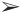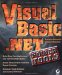# Accessing Excel

 Team Fly

Page 111

### Accessing Excel

Use ProjectAdd Reference, then click the COM tab and add the Microsoft Excel 10.0 Object Library (yours might be 9.0 or some other version number).

As you probably realize by now, if you've read this chapter, your first job when accessing Excel objects is to declare an application object:

` Dim exl As New Excel.Application `

Then you contact the primary unit of organization in Excel, the range object (which can be as small as a single cell or as large as a worksheet). The remaining sections describe how to use handy features of Microsoft Excel in your VB.NET programs.

#### Evaluating Math Expressions

One useful feature that Excel has to offer us VB.NET programmers is its ability to evaluate mathematical expressions. Given that VB.NET includes lots of math functions, such as SIN, COS, and so on (if you first Imports System.Math), why would you need to use Excel? For one thing, Excel allows you to submit a string for evaluation, thereby making it relatively simple to permit users to enter expressions into your application and have them evaluated.

Listing 4.14 shows how you can calculate a SIN in VB.NET, while Listing 4.15 is an example that uses Excel to evaluate the same expression.

 LISTING 4.14: EVALUATING MATH EXPRESSIONS IN VB.NET Imports System.MathPrivate Sub Form1_Load(ByVal sender As System.Object, _ ByVal e As System.EventArgs) Handles MyBase.Load        Dim n As Double = 4.3444        n = cos(n)        MsgBox(n)   End Sub

 LISTING 4.15: EVALUATING MATH EXPRESSIONS IN EXCEL Private Sub Form1_Load(ByVal sender As System.Object, _ ByVal e As System.EventArgs) Handles MyBase.Load        Dim exl As New Excel.Application        Dim n As Double
 Team FlyVisual Basic .NET Power Tools
ISBN: 0782142427
EAN: 2147483647
Year: 2003
Pages: 178

Similar book on Amazon